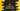# C program to find the average runs of cricket players using structure## c program to store the player info using structure and calculate the average runs of cricket players:

In this post, we will learn how to store the info of cricket players using structure and how to calculate the average run of these players using a C program.

With this program, you will learn how to use structures in C and how to create variables using structure, how to read data and store it in structure and how to do other calculations on these variables.

### Algorithm:

We will follow the below algorithm in this program:

• Define a structure to hold the cricketers data. It can hold the name and run of each cricketer.
• Ask the user to enter the total number of players and store it in a different variable.
• Create an array of structure that we created in the first step. This array will hold the name and run of each player.
• By using a loop, ask the user to enter the name and run of each player one by one.
• Store that in the structure variables.
• Run another loop, iterate through the array and find the average run.
• Print out the average run.

### C program:

Below is the complete C program:

``````#include <stdio.h>

struct player
{
int runs;
char name;
};

int main()
{
int n, i;
double sum = 0;

printf("Enter the total number of players: ");
scanf("%d", &n);

struct player p[n];

for (i = 0; i < n; i++)
{
printf("Enter the name of player %d: ", i + 1);
scanf("%s", p[i].name);

printf("Enter the total runs for player %d: ", i + 1);
scanf("%d", &p[i].runs);
}

for (i = 0; i < n; i++)
{
sum += p[i].runs;
}

printf("Average run: %.2f\n", sum / n);

return 0;
}``````

Here,

• n and i are two integer variables to hold the total number or players and to use in the loop. sum is a double variable to hold the total sum of runs. It is initailized as 0.
• player is a structure that can hold the runs and name of a player. runs is an integer variable and name is a character array.
• Ask the user to enter the total number of players. Read that value and store it in the variable n.
• Create an array of structure player to hold the details of the players.
• Run one for loop. This loop will ask the user to enter the detail of each player one by one. It will ask to enter the name and runs of each player and store that value in the structure array. We are using i to access the specific item in the array.
• Run one more for loop. This loop will find the total sum of all runs of the players. It adds the sum and stores it in the sum variable.
• At the end of the program, print the average of runs, i.e. divide the total sum by total number of player.

### Sample output:

If you run this program, it will print output as like below:

``````Enter the total number of players: 5
Enter the name of player 1: Alex
Enter the total runs for player 1: 121
Enter the name of player 2: Bob
Enter the total runs for player 2: 30
Enter the name of player 3: Chandler
Enter the total runs for player 3: 45
Enter the name of player 4: Donald
Enter the total runs for player 4: 50
Enter the name of player 5: Albert
Enter the total runs for player 5: 55
Average run: 60.20``````